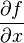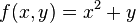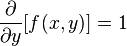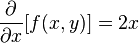# Partial derivative facts for kids

Kids Encyclopedia Facts

In calculus, an advanced branch of mathematics, the partial derivative of a function is basically the derivative of one named variable, and the unnamed variable of the function is held constant. In other words, the partial derivative takes the derivative of certain indicated variables of a function and doesn't differentiate the other variable(s). The notation$\frac{\partial f}{\partial x}$

is usually used, although other notations are not invalid. Usually, although not in all cases, the partial derivative is taken in a multivariable function, (i.e., the function has three or more variables, whether independent or dependent variables).

## Examples

If we have a function$f(x, y)=x^2+y$, then there are several partial derivatives of f(x, y) that are all equally valid. For example,$\frac{\partial }{\partial y}[f(x, y)]=1$

Or, we can do the following$\frac{\partial}{\partial x}[f(x, y)]=2x$

## Related pagesPartial derivative Facts for Kids. Kiddle Encyclopedia.